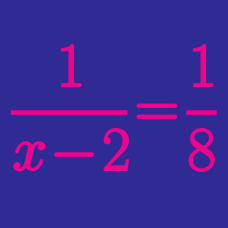Algebra

# Rational Functions: Level 2 Challenges

True or false:

All rational functions have some form of asymptote, whether it's slant, vertical, or horizontal.

How many vertical asymptotes are there on the following rational function?

$f(x) = \frac{x^{356} - 123x^{22} - 43x^{2}+10123}{x}$

$f(x) = \dfrac{(x-1)(x-2)(x-3)(x-4)(x-3)(x-2)(x-1)}{(x-2)(x-4)(x-2)}$

How many values of $x$ satisfy the equation $f(x) = 1$ ?

$f(x)=\dfrac{x^2-6x+6}{2x-4}$ $g(x)=\dfrac{ax^2+bx+c}{x-d}$

You are given two functions $f$ and $g$ above, where $a, b, c,$ and $d$ are unknown constants. Also, you are given the following information about the function $g$:

• It has the same vertical asymptote as $f$.

• Its diagonal asymptote is perpendicular to that of $f$, and these two asymptotes intersect each other on the $y$-axis.

• The graphs of $f$ and $g$ have two intersection points. One of them is at $x = -2$. (In other words, $f(-2) = g(-2)$.)

What is the value of the other $x$-coordinate where $f$ and $g$ intersect?

Let $x$ be a real number. Consider the function

$f(x) = \frac{x^3 - 1}{x^2 + x - 2}$

How many real zeroes does it have?

×